##### Formula and Execution
 Property value The current market value of the property is called property value. Annual gross income The revenue from the property in the shape of rent is called annual gross income. Operating expenses The day to day expenses of any business is called operating expenses. Vacancy rate The percentage of property which is still not rented out is called vacancy rate. Annual net income When operating expenses and vacancy rate is subtracted from gross income, the remaining amount is called annual net income. Cap rate The percentage of annual net income to property value is called Cap rate.

# Cap Rate Calculator

Surplus of money, when keeping idle, gives you no benefit but losing its value with each passing day. For that very reason, peoples invest their excess funds to gain some returns in the future. There are many sectors where investors can invest their money. Depend upon the level of risk each industry has, investors, invest their money accordingly in each industry. One such sector is the real estate sector, where investors can invest their money and get healthy and stable returns. There are different methods to calculate the rate of return on investment in the real estate sector. One such method is the capitalization rate, also called the Cap rate. Calculating the cap rate manually is a demanding and exhaustive task. “Calculator beast” has developed different tools to make the process of calculation easy and straightforward. One such tool is the capitalization rate calculator, also called the Cap rate calculator.

## Why is Cap rate calculator used?

The manual calculation has some key problems such as:

• Human is more vulnerable to errors as compared to a machine, i.e., calculations through the calculator make the result more reliable and complete as compared to manual calculations.
• Calculating the Cap rate manually is a hectic and time-consuming process.
• Manual calculation of Cap rate consumes much human energy and makes a person dull.

To get rid of such problems, we use the Cap calculator.

Cap calculator is convenient in many ways, such as:

• It gives you the error-free value of the Cap rate, subject to the condition that the values are correctly put in the calculator.
• It gives you quick calculation, so it saves a lot of your time.
• The calculation process of the Cap calculator is incisive and saves a lot of your energy.

The layman who doesn't know the formula of capitalization rate can also use this calculator to find the Cap rate.

### To whom will it help?

It is helpful for all those who need to calculate the capitalization rate on real estate investment, such as:

Property dealers: Those property dealers who purchased the building and then rent it out. They need to calculate the Cap rate to find out when they can recover the invested amount and start making a profit.

Researchers:  Those researchers who are researching investment in real estate need a Cap rate calculator for calculation of capitalization rate.

Students:  Those students who are doing their assignment on the capitalization rate. They can also require a Cap rate calculator to calculate the capitalization rate.

## Capitalization Rate

Cap rate is the rate of return which an investor is expecting to be generated by investment in real estate. The capitalization rate is measured based on the net income generated by the property in the shape of rent. The capitalization rate is a good indicator to compare the different rates of returns on rental property.

### Condition in Capitalization rate

While calculating the capitalization rate, the following conditions must be fulfilled.

• The rate of return must be calculated for not less than one year.
• The property must be purchased on cash.
• There must be no loan involved in the purchase of the property.

While calculating the capitalization rate, use the current market value of the property, not the purchase price of the property.

## Steps involved in Cap Rate Calculator

The Cap rate calculator operates in two modes. 1) Simple mode 2) Advance mode

### Simple mode

There are five steps involved in the calculation of Cap rate. These steps are:

1. Property value: This is the fundamental step in the calculation of the Cap rate, without putting the market value of the property, the Cap rate calculator gives no value of Cap rate.
2. Annual gross income: The value of annual gross income is also necessary for the calculation of the capitalization rate. Without the value of annual gross income, the cap rate calculator shows no value in the Cap rate box.
3. Operating expenses: This is an optional step if there is any value of operating expenses put that value otherwise leave it vacant.
4. Vacancy rate: This step is also optional if there is any value of the vacancy rate put that value otherwise leave it as it is.
5. Annual net income: Annual gross income and annual net income will be the same if the values of operating expenses and vacancy rates are zero. Otherwise, the net annual income will show a different amount, if operating expenses and the vacancy rate has any values other than zero.

Example: An individual purchases a property worth \$100,000. After one year, the market value of the property increases to \$120,000. The potential annual rental income from the property is \$20,000. The operating expenses are 5%, and the vacancy rate is 2%. Find the capitalization rate.

Solution:

Market value of the property = \$120,000.

Annual rental income = \$20,000

Operating expenses = 5%

Vacancy rate = 2%

Capitalization rate =?

Putt these values in the Cap rate calculator; the cap rate is obtained as follows.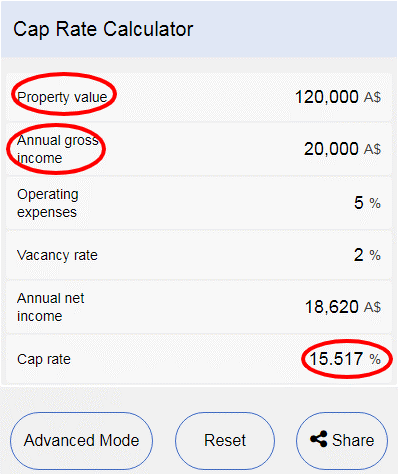Putting values in property value and annual gross income boxes is compulsory.

Note: We subtract the value of the vacancy rate from the annual gross income.

Reason: The reason behind the subtraction is that that vacancy rate shows the unrented part of the property; that's why we subtract the value of the vacancy rate.

Example: In the above example, the potential annual rental income is 20,000. But the whole of the property is not rented out; in fact, 2% of the property area is not rent out. So subtract that from the potential income, it gives us the real annual income i.e. 20,000 – 400 =\$19,600 (20000*0.02 = 400). 19,600 is an effective annual rental income. It means that the property has the potential to generate \$20,000 annual income, but in actual 2% of the property is not rented out, so when adjusted for this 2%, we get an effective annual income.

There is no such difference between the functions of advance mode and the simple mode. The only difference between the two is that that in advance mode, operating expenses amount is shown along with the percentage of operating expenses, as shown in the below picture.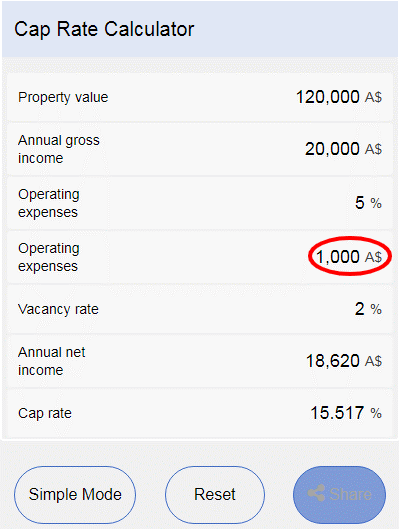### Formulas used in the Cap rate calculator

There are three formulas used in the capitalization rate calculator.

1. Vacancy rate formula: This formula is used to find the percentage of the property left unrented. The formula of the vacancy rate is (Unrented units of the property/ total units of the property) * 100.
2. Annual net income formula: This formula is used to find the effective or real rental income of the property. The formula of annual net income is (Gross income – operating expenses – vacancy rate).
3. Capitalization rate formula: This formula is used to find the rate of return on the investment in real estate. The formula of capitalization rate is (Annual net income/ Market value of the property)*100.

Example: An individual purchases an apartment building worth \$2,000,000. The potential annual gross income from the property is \$100,000. The operating expenses are 6% of the gross income. The building has 200 units in total, 190 are rented out, and ten are still unrented. Find vacancy rate, Annual net income, and capitalization rate.

Solution:

Property value = \$2,000,000

Annual gross income = \$100,000

Operating expense = 6%

Vacancy rate =?

Annual net income =?

Capitalization rate =?

Vacancy rate = unrented units / total units

= 10/200 = 0.05 or 5%

Vacancy rate = 5%

Annual net income = (Gross income – operating expenses – vacancy rate)

= (100,000 – 0.06*100,000 – 0.05*100,000)

= (100,000- 6,000- 5,000)

Annual net income = \$89,000

Capitalization rate = Annual net income / property value

= 89,000/ 2,000,000

Capitalization rate = 4%

Calculating capitalization rate manually is hectic and time consuming task. You can quickly solve it through Cap rate calculator as follow.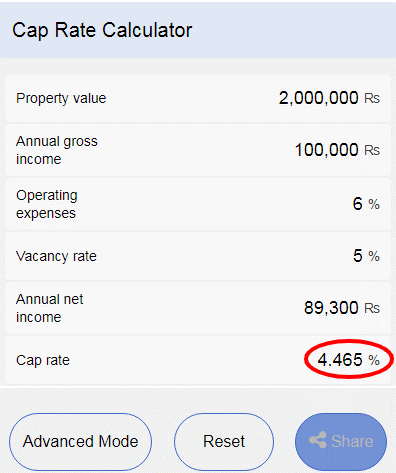The cap rate calculator gives you accurate and precise values.

If the market value of the property is not known i.e.

Annual gross income = \$100,000.

Cap rate = 4%

The market value of the property =?

Put these values in the cap rate calculator; it gives you the market values of the property as follows.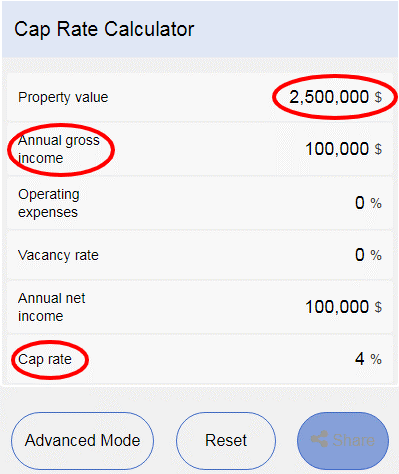If the annual gross income is unknown i.e.

Property value = \$2,000,000

Cap rate = 4%

Annual gross income =?

Put the above values in the cap rate calculator; it gives you the amount of annual gross income, as shown below.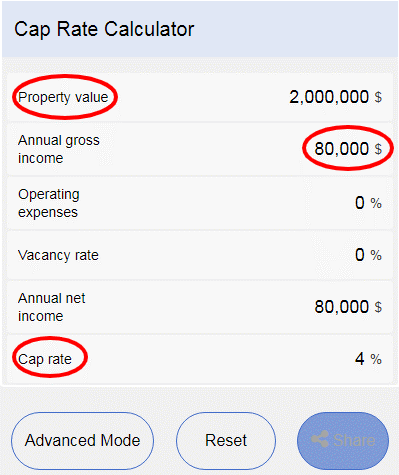## FAQS

### 1. What are the drawbacks of the capitalization rate?

The capitalization rate is a useful measurement of finding the annual rate of returns on real estate investment. But it has some drawbacks like:

• The capitalization rate does not consider the time value of money.
• The Cap Rate is not considering the loan amount borrowed for the purchase of the property.
• It does not consider the future inflow of cash as a result of improvement in the property.

### 2. The current market value of the property or purchase price of the property, which one is best to be used in the capitalization rate formula?

For the computation of the capitalization rate, several versions of the cap rate formula exist. Some real estate expert uses the current market value of the property. In contrast, others use purchase price or the historical cost of the property in the cap rate formula.

1. Capitalization rate =Annual net income / Current market value of the property.
2. Capitalization rate =Annual net income/ Acquisition cost of the property.

The first formula is widely used because it uses the prevailing market value of the property and takes into account the value added to the property over the period.

The problem with the use of the second formula is that it uses the historical value of the property. We know that changes occur in the market prices of the property. If we use the historical cost of the property in the calculation, it doesn't take the changes in property prices into account, which makes the Cap rate less reliable. That’s why using the current market value of the property in calculation of capitalization rate gives us the most accurate and logical rate of returns on real estate investment.

### 3. How can we solve the capitalization rate in excel?

There is no specific function in excel for Cap rate calculation, but we can solve it manually as follow.

• Put the current market value of the property in any cell of the Ms excel.
• Put the amount of the gross income in the subsequent cell.
• Insert the value of operating expenses, if any, in the following cell.
• Put the value of the vacancy rate, if any, in the next cell.
• Subtract operating expenses and vacancy rate if any from the gross income, it gives us net income.
• Apply the formula of capitalization rate, i.e. (Net income / Market value of the property); it provides us with the cap rate as follows.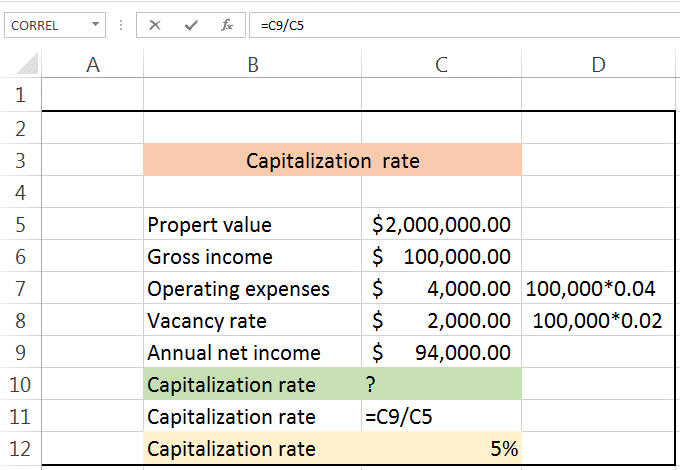Note: Put ‘=’ before applying formula like in the picture above. Without ‘=’ sign before formula, Ms excel will not apply the function.

Remember: In the above question, operating expenses are 4% of the gross income, and the vacancy rate is 2% of the gross income.

### 4. On how many factors cap rate depends?

The Cap rate depends on four main factors. These factors are:

1. Annual net income
2. Value of the property
3. Operating expenses
4. Vacancy rate

Keeping the other factors constant, the value of the Cap rate increases with the increase in the net yearly income. Keep the other factors constant; the cap rate value decreases with the increase in the property value. There is a direct relation between Cap rate and annual net income when the amount of annual net income increases the Cap rate increases and vice versa. An inverse relationship exists between property value and the Cap rate. When property value increases, the Cap rate decreases, and vice versa.

### 5. What is a good and bad cap rate?

There are no good and bad Cap rates; it depends on the investment you have done. Cap rate depends upon the market conditions, location of the property, type of property. For some, a cap rate of 8% is reasonable, while for others, it is a bad cap rate. So Cap rate is a relative term, and there are no good and bad Cap rates

### 6. Can I use the cap rate in business valuation?

Yes, the Cap rate can be used in discounting free cash flows, dividend discount model, and other valuations of the business.

### 7. What is the 2% rule in real estate?

This rule states that an investment in real estate is a good investment if the rental income from the real estate investment is 2% of the investment. For example, a person invested \$100,000 in real estate; he must earn at least \$2,000 in rental income to consider his investment a good investment. But this rule is not prevailing in the real estate market, so this 2% is not a standard with which you can compare the failure or success of the investment.

### 8. Is the Cap rate and ROI the same?

ROI stands for return on investment. There is a difference between the two.

In Cap rate, the property purchased is a pure cash base. There is no involvement of installments or loans, i.e., the property purchased is purely on personal cash.

While in ROI, The property purchased involves some cash base transaction along with a loan, i.e., the property is purchased by the combination of a loan and personal cash.

The calculation process of both the methods is the same; only the nature of the transaction involved is different.

### 9. What is the 70% rule?

70 % rule is used in flipping the property. Flipping the property means that you purchase a property at a low price, then repair it and sale on a higher rate.

According to the 70% rule, while purchasing property, The investor should not pay more than 70% of the after repair value.

Example: The after repair value of the property is \$200,000, and the repairing cost is \$10,000. According to 70% rule the investor should pay \$140,000 I.e. (200,000*0.7= 140,000) to buy the property. The investor will earn \$ 50,000 i.e. (200,000 – 140,000 – 10,000) from this business transaction.

### 10. What is the 50% rule?

This rule states that your expenses should not exceed 50% of your rental income. The expenses include operating expenses. The operating are insurance charges, legal charges, maintenance charges.

Example: A property worth \$200,000, is generating \$40,000 annual rental income. The operating expenses are \$15,000. According to the rule operating expenses should not exceed 50% of the total rental income. Now let's check it.

Rental income = \$40,000

50% of the rental income = 40,000* 0.5 = \$20,000

The operating expenses are \$15,000, while the allowed margin is \$20,000. So the operating expenses are in the allowed bracket.

### 11. Does Cap rate include mortgage?

Cap rate doesn't account for the mortgage payment, as you can see in the formula of Cap rate formula. Cap rat only consider operating expenses and vacancy rate in its calculation. So Cap rate does not include mortgage in the calculation.

### 12. Is property tax included in Cap rate?

The operating expenses which are deducted from the gross income while calculating the Cap rate include tax amount. So yes, property tax is included in Cap rate.

### 13. Does Cap rate include depreciation?

As depreciation is a non-cash expense, and Cap rate calculation does not include any non-cash costs. So depreciation is not included in Cap rate.

### 14. What is an exit Cap rate?

An exit Cap rate is also called the terminal Cap rate. This is an estimated Cap rate on the date of disposal of the property. It is calculated as (Expected net income / expected sale price of the property)*100.

### 15. What does high and low cap rates mean?

According to a theory of finance, the higher the risk higher will be returns and vice versa. If the Cap rate is high, then it means that the property is risky and vice versa.

### 16. When not to use the Cap rate?

The Cap rate does not make any sense in the following scenarios.

1. Fix and flip: In case of fix and flip of the property, Cap rate calculation does not make any sense because, in this case, the property is held for selling purpose. There are no intentions of renting the property, so that's why the Cap rate calculation does not make any sense.
2. Purchasing a vacant property: Cap rate depends on two main factors, I.e., net annual income and value of the property. In the case of vacant property, the rental income amount is not available, and it is complicated to estimate rental income and operating expenses. So capitalization rate does not make any sense in case of a vacant property.
3. Short term rental property: Cap rate is calculated for at least one year. In the case of short term rental property where the rental duration is less than one year, the capitalization rate calculation does not make any logical sense.

### 17. What are the factors that affect the cap rate?

Higher or lower cap rate depends upon many factors such as:

• Location: A property in a decent area increases the demand for the property. As a result of higher demand, the rental income also increases and hence rise in Cap rate.
• Asset class: The type of property also plays a vital role in the higher and lower Cap rate. Generally, commercial properties have high rates as compare to residential properties.
• Interest Rates: The Cap rate is inversely related to the interest rate. Higher the interest rate lower will be the Cap rate. The reason behind this inverse relationship is that that in time of higher interest rates, the investor will divert their investment into government securities and other financial instruments of the banks. This diversion of funds will lower the demand for investment in the real estate sector and hence lower cap rate.